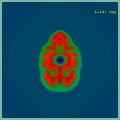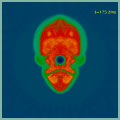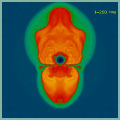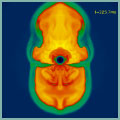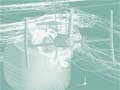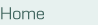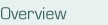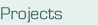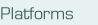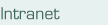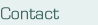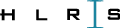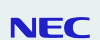# Projects

### PROMETHEUS/VERTEX

PROMETHEUS/VERTEX is a multidimensional neutrino radiation hydrodynamics code which was developed at MPA to simulate supernova explosions of massive stars.

For the supernova problem an accurate computation of the energy and lepton number source terms due to neutrino heating is essential. Hence, the Boltzmann equation of neutrino transport needs to be solved together with the hydrodynamics. Since the transport problem requires:

• the solution of a highly dimensional problem in neutrino phase space per time step
• implicit discretization to allow for sufficiently large time steps a computational performance beyond a TFLOP/s is necessary for this type of simulation.

#### Numerics

On a radial ray, angular moment equations of the Boltzmann equation are discretized with backward time differencing, giving rise to a non-linear algebraic system which is solved with a Newton-Raphson procedure. Within a Newton-Raphson step, inversions of a block-pentadiagonal matrix with dense blocks are required. A similar Newton-Raphson procedure is employed for solving a model Boltzmann equation. Both solutions are iterated to consistency on each ray.

The entire code has been vectorized. Parallelization is performed at a coarse level over the different radial rays. A mixed OpenMP/MPI programming model has been used to assign radial rays to different CPUs.

#### Simulation Results

The figure shows the snapshot from a simulation of the evolution of the neutrino heated supernova shock in a 11.2 solar mass star. The simulation was performd using a full 180 degree computational grid.
A weak explosion with a prolate shock developed.
This low-resolution simulation required about half a year of computation on a 32 CPU IBM p690.

So far, the supernova problem is still unsolved. The enormous computational requirements have defied parameter studies for identifying the crucial piece of physics in a successful supernova simulation. Substantial progress in the field is expected from TFlop simulations, which will allow to treat the neutrino transport more accurately, and to vary uncertain input physics in the simulations.

#### Contact

Konstantinos Kifonidis
Max-Planck-Institut für Astrophysik
Phone: ++49-89-30000-2245
Fax: ++49-89-30000-2235
E-Mail: kok@mpa-garching.mpg.de

Hans-Thomas Janka
Max-Planck-Institut für Astrophysik
Phone: ++49-89-30000-2228
Fax: ++49-89-30000-2235
E-Mail: thj@mpa-garching.mpg.de